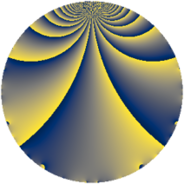# Properties

 Label 3549.2.cLevel $3549$ Weight $2$ Character orbit 3549.c Rep. character $\chi_{3549}(337,\cdot)$ Character field $\Q$ Dimension $152$ Sturm bound $970$

# Related objects

## Defining parameters

 Level: $$N$$ $$=$$ $$3549 = 3 \cdot 7 \cdot 13^{2}$$ Weight: $$k$$ $$=$$ $$2$$ Character orbit: $$[\chi]$$ $$=$$ 3549.c (of order $$2$$ and degree $$1$$) Character conductor: $$\operatorname{cond}(\chi)$$ $$=$$ $$13$$ Character field: $$\Q$$ Sturm bound: $$970$$

## Dimensions

The following table gives the dimensions of various subspaces of $$M_{2}(3549, [\chi])$$.

Total New Old
Modular forms 512 152 360
Cusp forms 456 152 304
Eisenstein series 56 0 56

## Trace form

 $$152q - 148q^{4} + 152q^{9} + O(q^{10})$$ $$152q - 148q^{4} + 152q^{9} - 16q^{10} - 16q^{12} + 156q^{16} - 16q^{17} + 48q^{22} - 120q^{25} + 16q^{29} - 8q^{30} + 8q^{35} - 148q^{36} - 56q^{38} + 8q^{40} - 4q^{42} - 40q^{43} + 64q^{48} - 152q^{49} + 24q^{51} + 32q^{53} + 16q^{55} + 8q^{61} + 32q^{62} - 228q^{64} + 64q^{68} - 8q^{69} - 72q^{74} - 32q^{75} + 16q^{77} + 16q^{79} + 152q^{81} - 40q^{82} - 16q^{87} - 48q^{88} - 16q^{90} + 24q^{92} + 80q^{94} + 48q^{95} + O(q^{100})$$

## Decomposition of $$S_{2}^{\mathrm{new}}(3549, [\chi])$$ into newform subspaces

The newforms in this space have not yet been added to the LMFDB.

## Decomposition of $$S_{2}^{\mathrm{old}}(3549, [\chi])$$ into lower level spaces

$$S_{2}^{\mathrm{old}}(3549, [\chi]) \cong$$ $$S_{2}^{\mathrm{new}}(39, [\chi])$$$$^{\oplus 4}$$$$\oplus$$$$S_{2}^{\mathrm{new}}(91, [\chi])$$$$^{\oplus 4}$$$$\oplus$$$$S_{2}^{\mathrm{new}}(169, [\chi])$$$$^{\oplus 4}$$$$\oplus$$$$S_{2}^{\mathrm{new}}(273, [\chi])$$$$^{\oplus 2}$$$$\oplus$$$$S_{2}^{\mathrm{new}}(507, [\chi])$$$$^{\oplus 2}$$$$\oplus$$$$S_{2}^{\mathrm{new}}(1183, [\chi])$$$$^{\oplus 2}$$